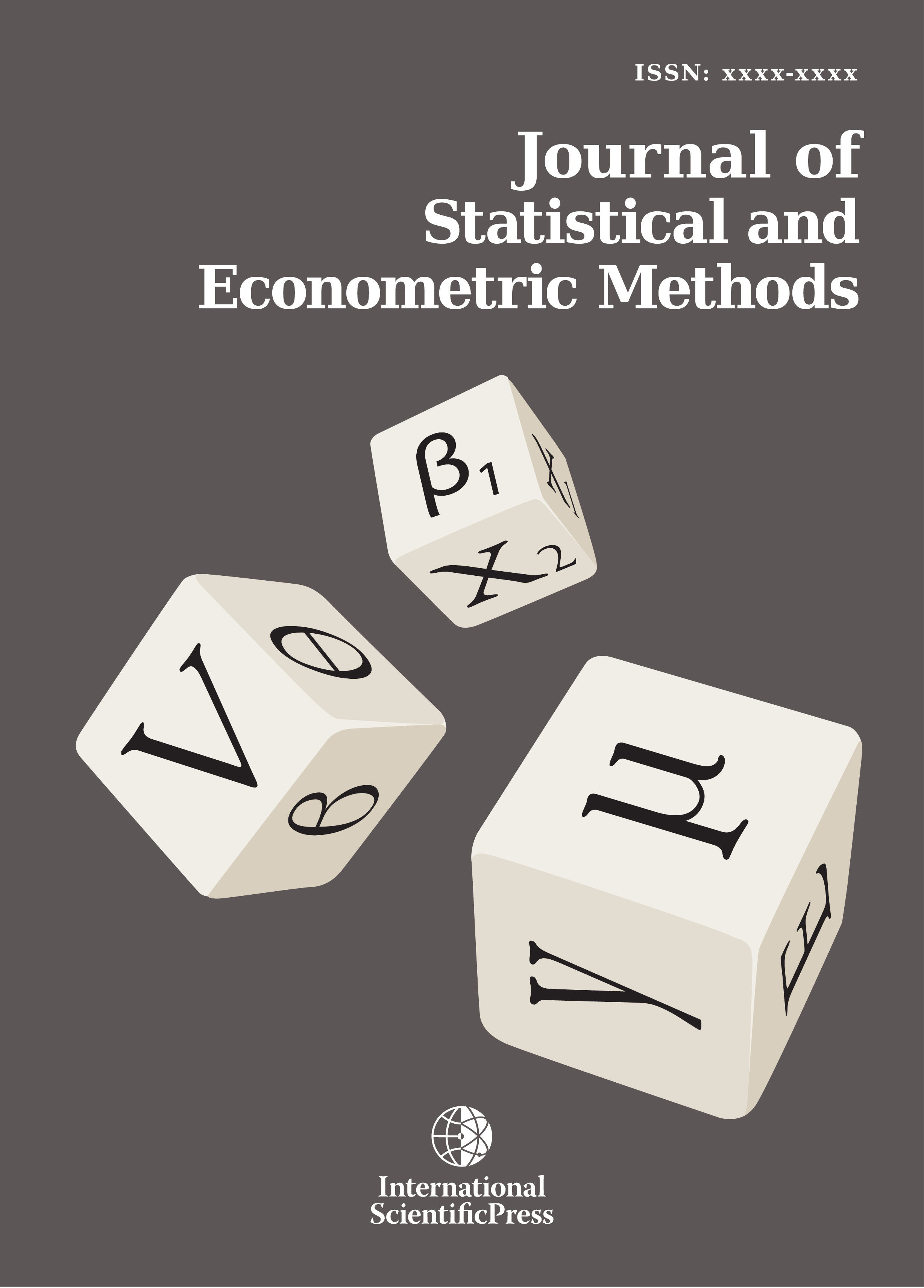# Journal of Statistical and Econometric Methods

#### Challenge the Approach of Collaboration of Statistical Methods in Selecting the Correct Multiple Linear Regressions with Violation of some Assumptions

•[ Download ]
• Times downloaded: 1045
• Abstract

The analysis of multiple linear regressions (MLR) is investigated in the current study. MLR is applied frequently in different field of applied studies. In the current study simulation technique is used to evaluate the approach of "The Approach of Collaboration of Statistical Methods in Selecting the Correct Regressions (ACSMSCR)" by AL-Marshadi, (2014) with its two options in terms of its ability to identify the right regression model under some model assumptions violations with two different sample sizes. The evaluation is in terms of the percentage of number of times of success in identifying the right model. The simulation results indicate that the approach of (ACSMSCR) provided very good choice to select the right model even under the considered model assumptions violations where the second option provided the best performance for both the sample sizes. The main result of the current study is that we suggest using the approach of (ACSMSCR) with the second option as a reliable procedure to select the right model even under the considered model assumptions violations.ISSN: 2241-0376 (Online)
2241-0384 (Print)